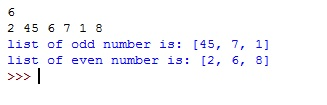# Python | Program to print Odd and Even numbers from the list of integers

In this article, we are going to find odd and even numbers from the given list of integers using Python program.
Submitted by Anamika Gupta, on July 17, 2018

Logic: To do this, we will simply go through the list and check whether the number is divisible by 2 or not, if it is divisible by 2, then the number is EVEN otherwise it is ODD.

Program:

```# Give number of elements present in list
n=int(input())

# list
l= list(map(int,input().strip().split(" ")))

# the number will be odd if on diving the number by 2
# its remainder is one otherwise number will be even
odd=[]
even=[]

for i in l:
if(i%2!=0):
odd.append(i)
else:
even.append(i)

print("list of odd number is:",odd)
print("list of even number is:",even)
```

OutputPreparation